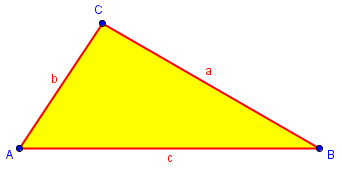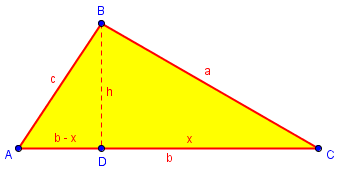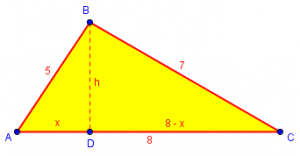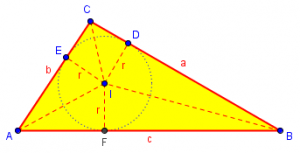# Area of a Triangle: Heron’s Formula I

There is a beautiful formula for the area of a triangle, which many students unfortunately never get to see. In this post we’ll look at that formula and three ways to prove it; next time, I’ll show some examples of how useful it can be. (By the way, you’ll also see it called “Hero’s Formula”, based on a different translation of its Greek creator’s name.) Eventually in this series, we’ll get to some very practical problems about finding area.

## The formula

Here is a good introductory question, from 1995 (when Ask Dr. Math was less than a year old):

The Area of Triangles using Hero's Formula

I have been surfing for about a week and this is the first site I have visited that actually had an area like this.  I am currently producing (trying) a software package for Facilities Management.

Question.
If a person gave three dimensions of a triangle (in feet) and at least noted the Base dimension, without knowing the angles because the other two lines would have to intersect someplace, is there a formula that could calculate the area?  Dimension of a roof area for example up to five measurements, squares, rectangles and odd size rectangles ok, but I am having a problem with the other two. When you ask for the height of a triangle you are met with a "what?". Pentagons knowing only the five dimensions?

Thank you for the opportunity to at least submit this. Just trying to present the problem in written form helps sometimes.

Hello!

Here's one of my favorite formulas in Math: it's Hero's formula (sometimes called Heron's formula) for the area of a triangle.  If the 3 side lengths are a, b, and c, then let s = (a+b+c)/2.  The s stands for semiperimeter.  Then the area of the triangle is

Sqrt{s(s-a)(s-b)(s-c)}.

As far as your pentagon question, I'm not sure I understand what you mean.  Are you given only the five side lengths?  If so, then you can't figure out what the area is from that information alone (a pentagon isn't a rigid figure in the same way that a triangle is).

As you may know from the SSS congruence theorem, a triangle is fully determined by the lengths of its sides, so that’s all you need to find the area. That is not true for polygons with more sides. Later, we’ll be looking at ways to find such areas, some of which involve breaking them into triangles and using Heron.

The formula is one that is easily memorized, though not easy to prove (and therefore one of the few that I have to memorize rather than recreate it when needed).

Here is our triangle, with sides a, b, and c:We first calculate the semiperimeter (half the sum of the sides): $$s = \frac{a+b+c}{2}$$. Then the area of the triangle is $$K = \sqrt{s(s-a)(s-b)(s-c)}.$$

If we replace s in the formula with its definition, we get this form, which is a little less memorable but equally interesting: $$K = \sqrt{\frac{a+b+c}{2}\cdot\frac{-a+b+c}{2}\cdot\frac{a-b+c}{2}\cdot\frac{a+b-c}{2}}.$$

So, why would this work? We’ve been asked that a few times, so here are three proofs: one using trigonometry, one using the Pythagorean Theorem, and one using circles and triangles. If you don’t follow one proof, try the next. The second will show a way I often work around the formula for those who don’t know it, so it’s useful beyond being a proof.

## Proof #1: Law of Cosines

First, a question from 1997:

Proof of Hero's formula

Could you tell me where to find a proof of Hero's formula or help on how to derive it?  I am a teacher and my class is working on it.

Doctor Anthony answered using the Law of Cosines, together with double-angle formulas for both sine and cosine.

Recall that the Law of Cosines says that $$a^2 = b^2 + c^2 – 2bc\cos{A},$$ so that $$\cos{A} = \frac{b^2 + c^2 – a^2}{2bc}.$$ The double-angle formulas are $$\sin(2x) = 2\sin(x)\cos(x)\\ \cos(2x) = \cos^2(x) – \sin^2(x) = 2\cos^2(x) – 1 = 1 – \sin^2(x).$$

(He uses a period for multiplication.)

We use the formula

area = (1/2)bc.sin(A)

And sin(A) = 2 sin(A/2).cos(A/2)

so area = bc.sin(A/2).cos(A/2)

Next we find expressions for cos(A/2) and sin(A/2) in terms of s, a, b, c, where a, b, c are the sides of the triangle and s is the semi-perimeter.

So  s = (a+b+c)/2

b^2 + c^2 - a^2
cos(A) = 2cos^2(A/2) - 1 = ---------------
2bc

b^2 + c^2 - a^2
2cos^2(A/2) = 1 + ---------------
2bc

(b+c)^2 - a^2   (b+c-a)(b+c+a)
= ------------- = --------------
2bc             2bc

(2s-2a)2s
= ---------
2bc

s(s-a)
cos^2(A/2) = ------
bc

Next cos(A)   = 1 - 2 sin^2(A/2)
b^2 + c^2 - a^2
= ---------------
2bc

b^2 + c^2 - a^2
2sin^2(A/2) = 1 - ----------------
2bc

a^2 - (b-c)^2   (a-b+c)(a+b-c)
= ------------- = --------------
2bc             2bc

(2s-2b)(2s-2c)
=  --------------
2bc

(s-b)(s-c)
sin^2(A/2) = ----------
bc

We can now return to the formula for the area of the triangle:

(1/2)bc.sin(A) = bc.sin(A/2).cos(A/2)

bc.sqrt[s(s-a)(s-b(s-c)]
= ------------------------
bc
= sqrt[s(s-a)(s-b)(s-c)]

It isn’t obvious how you would know to do this (that’s true of many proofs in their final form), but it’s beautiful how the parts of the formula fall into place two by two!

## Proof #2: Pythagorean Theorem

A year later, we got this question:

Derivations of Heron's Formula

I understand how to use Heron's Theory, but how exactly is it derived?

Doctor Rob referred to the proof above, and then gave one that I tend to use:Another proof uses the Pythagorean Theorem instead of the trigonometric functions sine and cosine. Drop from B a perpendicular to side b. Let its length be h. Let the side b be divided by it into parts of lengths x and b - x. Then using the Pythagorean Theorem on the two small triangles, and the usual formula for area of a triangle:

a^2 = h^2 + x^2
c^2 = h^2 + (b-x)^2
K = b*h/2

Then by subtracting the second equation from the first:

a^2 - c^2 = 2*b*x - b^2
x = (a^2+b^2-c^2)/(2*b)

Substituting this back into the first equation:

h^2 = a^2 - (a^2+b^2-c^2)^2/(4*b^2)

Now using the third equation:

K^2 = b^2*h^2/4
= (b^2/4)*[a^2 - (a^2+b^2-c^2)^2/(4*b^2)]
= a^2*b^2/4 - (a^2+b^2-c^2)^2/16
= (4*a^2*b^2-a^4-2*a^2*b*2-b^4+2*b^2*c^2-c^4+2*a^2*c^2)/16
= -(a^4+b^4+c^4-2*b^2*c^2-2*a^2*c^2-2*a^2*b^2)/16
= -(c^4 + [-2*b^2-2*a^2]*c^2 + [a^4-2*a^2*b^2+b^4])/16

We factor this by completing the square on c^2, then using the difference of two squares three times, then a + b + c = 2*s:

K^2 = -(c^4 + [-2*b^2-2*a^2]*c^2 + [a^2+b^2]^2 - 4*a^2*b^2)/16
= -([c^2-a^2-b^2]^2-[2*a*b]^2)/16
= -([c^2-a^2-2*a*b-b^2]*[c^2-a^2+2*a*b-b^2])/16
= -(c^2-[a+b]^2)^2*(c^2-[a-b]^2)/16
= -(c+[a+b])*(c-[a+b])*(c+[a-b])*(c-[a-b])/16
= (a+b+c)*(a+b-c)*(a-b+c)*(-a+b+c)/16
= (2*s)*(2*s-2*c)*(2*s-2*b)*(2*s-2*a)/16
= s*(s-a)*(s-b)*(s-c)
K = sqrt[s*(s-a)*(s-b)*(s-c)]

The algebra got pretty hairy there, though the basic idea is simple. Let me demonstrate how this method works as a calculation in a particular case, for those who haven’t learned (or forget) the formula:

Suppose we know that the sides of a triangle are 5, 7, and 8, and want to find the area.

First, we can use Heron’s formula, using s = 10: $$K = \sqrt{10\cdot 5\cdot 3\cdot 2} = \sqrt{300} = 10\sqrt{3}.$$

If we don’t know the formula, we can do what we did in this proof (I’ve swapped the position of x to what feels more natural to me):We can write an equation that says h is the same in each small triangle: $$5^5 – x^2 = 7^2 – (8-x)^2.$$ Solving this for x, we find that $$x = \frac{5}{2}$$. (It turned into a linear equation, very easy to solve.)

Applying the Pythagorean Theorem to either triangle, we find that $$h = \frac{5}{2}\sqrt{3}$$.

Finally, the area is $$\frac{1}{2}bh = \frac{1}{2}\cdot 8\cdot \frac{5}{2}\sqrt{3} = 10\sqrt{3}.$$

That’s much simpler than proving the formula, but a little harder than using it.

For an alternative approach, you could write an equation that says AD + DC = 8, and solve directly for h; but that is a radical equation that most of us would rather avoid! (I’ll be demonstrating it next time, though.)

## Proof #3: Inscribed circles

Finally, in 2000 we got a request for a purely geometric proof:

Geometric Proof of Heron's Formula

How do I prove Hero(n)'s formula using a circle with center P and radius R inscribed in triangle ABC?

Doctor Floor took this one (though his answer may not be what the hint was intended to lead to). Note that an incircle, or inscribed circle, is (internally) tangent to the sides of triangle, while an excircle is tangent to one side and to the extensions of the other two sides.

I know a method to prove Heron's formula geometrically with the help of the incircle of the triangle, but in this method I also use one of the excircles. I learned this method from Paul Yiu of Florida Atlantic University.

First, I have made a picture for you of the triangles and the two circles. Note that I have changed names: the incircle has center I and radius r (while the excircle (opposite to A) has center I' and radius r') instead of your center P and radius R.Take a moment to orient yourself; find triangle ABC, and the centers of the two circles.

In the following, the sides of the triangle are written as a = BC, b = AC, and c = AB. I use s = (a+b+c)/2 for the semiperimeter.

1. From the fact that two tangents to a circle are congruent, we see that AE = AG, CG = CF and BE = BF. So, for instance:

AE+EB+CG = c+CG = s

and thus CG = s-c.

In the same way:

CF = s-c
AG = AE = s-a
BE = BF = s-b

We’re already starting to see the formula take shape, in the form of the three differences.

2. Again from the fact that two tangents to a circle are congruent we see that AE' = AG, BE' = BJ and CG' = CJ. This gives us:

AG'+AE' = AB+BJ+CJ+AC = 2s

And we can conclude that AG' = AE' = s. And, for instance,
BE' = s-c.

These are popping up all over the place. But we still don’t know any areas.

3. Both I and I' lie on the internal angle bisector of <A.

I' lies also on the external angle bisectors of <B and <C. These external angle bisectors are perpendicular to the respective internal angle bisectors. So BI and BI' are perpendicular; CI and CI' are perpendicular as well.

4. From step 3 we can conclude that triangles EBI and E'I'B are similar. From this we can conclude from E'I'/E'B = EB/EI:

r'   s-b
--- = ---
s-c    r

and thus

r*r' = (s-b)(s-c)   ....................

The pieces are coming together!

In this picture, the yellow triangles are the pair used above, and the green are used next:5. We can also see that triangles AIG and AI'G' are similar. Here we can conclude from IG/I'G' = AG/AG' that:

r    s-a
-- = ---   .............................
r'    s

6. Multiplying  and  gives:

(s-a)(s-b)(s-c)
r^2 = ---------------
s

and

(s-a)(s-b)(s-c)
r = sqrt(---------------)   ............
s

Now we have a formula for the inradius r of ABC. Look at this close-up of ABC:The areas of triangles AIB, BIC, and CIA are respectively $$\displaystyle\frac{cr}{2}$$, $$\displaystyle\frac{ar}{2}$$, and $$\displaystyle\frac{br}{2}$$, so their sum, the area of ABC, is $$\displaystyle K = \frac{ar}{2} + \frac{br}{2} + \frac{cr}{2} = \frac{a+b+c}{2}r = sr$$. So …

7. It is not difficult to see that the area of ABC, let's call it K, equals s*r. Combining this with  we find Heron's formula:

K = sqrt(s(s-a)(s-b)(s-c))

And there’s the formula!

Next time, we’ll use it in some useful and surprising ways.

### 3 thoughts on “Area of a Triangle: Heron’s Formula I”

This site uses Akismet to reduce spam. Learn how your comment data is processed.